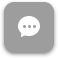Call Now 855-300-1469

MTH 1703 Finite Mathematics

Course Description

In this course, a student will be exposed to topics in mathematics that are especially applicable to business, such as linear models, linear programming, mathematics of finance, counting methods, probability, and statistics

Course Objectives

Upon completion of this course, students should be able to

• To prepare for courses of study in finance, statistics, quantitative methods, accounting, marketing, economics, and computer information systems
• Successfully answer questions pertaining to certain topics included in the CLAST exams
• Use quantitative reasoning to enhance decision-making capability

Week 1

Lecture: Set Theory

Outcomes

• Discuss the basic concepts of set theory
• Use three methods to represent sets
• Determine whether two sets are equal or equivalent
Lecture: Venn Diagram

Outcomes

• Discuss the meaning of a universal set
• Determine the number of subsets of a set
• Perform operations with sets
• Use Venn diagrams to illustrate equality of sets

Week 2

Lecture: Algebra Review

Outcomes

• Evaluate algebraic expressions
• Simplify algebraic expressions
• Evaluate formulas
Lecture: Linear Equations and Inequalities

Outcomes

• Solve linear equations
• Solve for a variable in an equation or formula
• Solve a “word” problem using linear equations
• Graph the solutions of an inequality on a number line
• Solve applied problems using linear inequalities

Week 3

Lecture: Graphs and Functions

Outcomes

• Graph equations
• Use the f(x) notation
• Use intercepts to graph a linear equation
• Calculate the slope of a line
• Interpret slope and y-intercept in applied situations
Lecture: Linear Systems

Outcomes

• Solve linear systems by graphing, substitution, and addition
• Solve “word” problems using systems of linear equations
• Graph a system of linear inequalities

Week 4

Lecture: Consumer Mathematics and Financial Management - Part 1

Outcomes

• Solve applied problems involving percent
• Calculate simple interest and maturity value
• Use compound interest formulas
• Calculate present value
• Understand and compute effective annual yield
• Find the value of an annuity
Lecture: Consumer Mathematics and Financial Management - Part 2

Outcomes

• Determine the amount financed, the installment price, and the finance charge for a fixed loan
• Compute unearned interest and the payoff amount for a loan paid off early
• Find the interest, balance due, and minimum monthly payment for credit card loans
• Compute interest costs for a mortgage
• Recognize stocks, bonds, and mutual funds as investments

Week 5

Lecture: Counting Methods and Probability Theory

Outcomes

• Use the Fundamental Counting Principle to determine the number of possible outcomes in a given situation
• Use the permutations formula
• Use the combinations formula
• Distinguish between permutation and combination problems
• Compute theoretical and empirical probability
• Compute probabilities with permutations
• Compute probabilities with combinations

Week 6

Lecture: Odds, Conditional Probability, and Expected Value

Outcomes

• Determine and use odds
• Compute conditional probabilities
• Use expected value to determine the average payoff or loss in a game of chance

Week 7

Lecture: Statistics

Outcomes

• Describe the population whose properties are to be analyzed
• Organize and present data
• Identify deceptions in visual displays of data
• Calculate the measures of central tendency
• Determine the standard deviation for a data set

Week 8

Lecture: Normal Distribution

Outcomes

• Recognize the characteristics of normal distributions
• Convert a data item to a z-score
• Identify and use percentiles
• Use and interpret margins of error

The course description, objectives and learning outcomes are subject to change without notice based on enhancements made to the course. November 2011